# Data Interpretation

``` (adsbygoogle = window.adsbygoogle || []).push({}); ```

``` (adsbygoogle = window.adsbygoogle || []).push({}); ```

## Data Interpretation Introduction

Interpretation of Data……that is all this section is about. The purpose here is to test a candidate’s ability to look at some data and make logical inferences from that.

Data is defined as

1. Factual information, especially information organized for analysis or used to reason or make decisions.
2. Computer Science. Numerical or other information represented in a form suitable for processing by computer.
3. Values derived from scientific experiments.

In general, data consist of propositions that reflect reality. Raw data are numbers, characters, images or other outputs from devices to convert physical quantities into symbols, in a very broad sense. Such data are typically further processed by a human or input into a computer, stored and processed there, or transmitted (output) to another human or computer. Raw data is a relative term; data processing commonly occurs by stages, and the “processed data” from one stage may be considered the “raw data” of the next.

Some examples of organized data are

1. Breakup of Indian population under various categories like people under the age of 16, above 16 but less than 25, above 25 but less than 40 etc.
2. Time table and occupancy chart of classroom teachers defining students of which standard shall be sitting in which classroom, and who will teach them
3. Software exports from India to various countries, in different months and the profitability of the Indian Majors in the given months etc.

Data Interpretation as the name implies is extraction of maximum information as required by us from the given set of data or information. Managements Entrance tests check your DI skills so as to check your ability to calculate fast, and comprehend relevant information. As managers of tomorrow, you will constantly come across different tables, pie-charts, bar-graphs, line-graphs etc., hence it is imperative that you must be skilled in DI.

Let us start with some simple examples –

See the graph below that shows the exchange rate variation between US Dollar and British Pound over a few months period.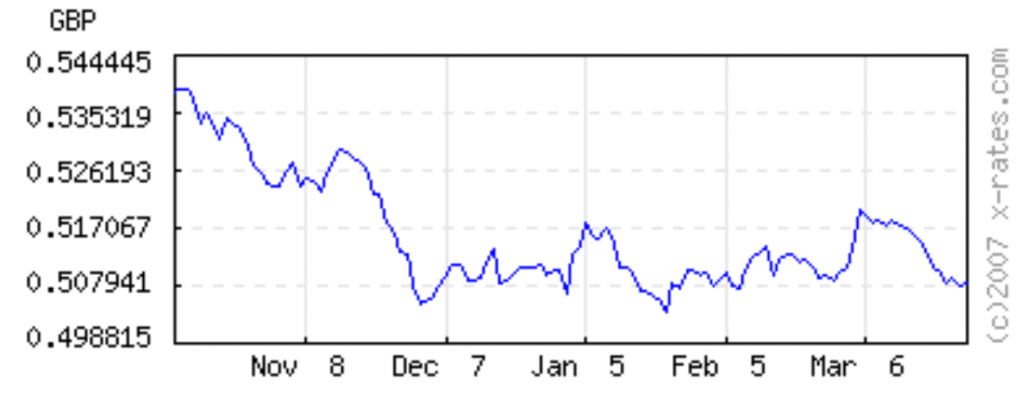Various questions can be asked from the graph above, e.g.

1. Has the dollar appreciated or depreciated vs Pound ?
2. Was it cheaper to buy a US dollar on Dec 7th or Mar 6th ?
3. If you had bought 100 US dollars on Nov 8th and sold them on Feb 5th, would you have gained or lost money and how much ?

The information for all the above questions is in the graph above, its just not written in plain English for you. The task here is to gather the right information from the graph, interpret it and then make decisions.

Lets try a different data format on the same currency exchange topic. The table below shows the exchange rate between different currencies on a certain recent date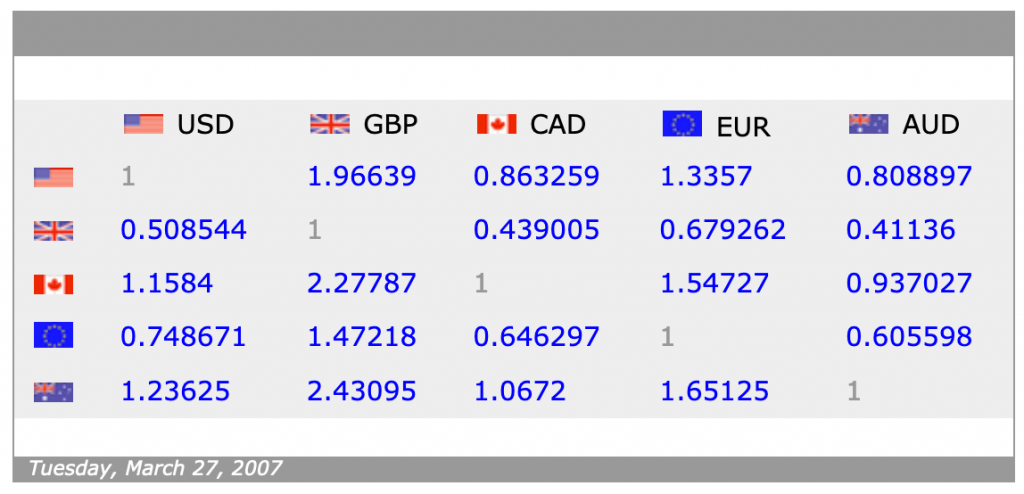1. If you have 100 GBP, can you buy more units of USD or EUROs ?
2. If you have 100 Pounds and 100 Euros, how many dollars do you have ?
3. Assuming that there are no service charges and the rates above work for both buying and selling, if you start with 100 Euros and
1. convert them into USD
2. then convert USD into AUD
3. then convert AUD into Euros

Do you end up with more / less / equal to 100 Euros ?

The data presentation would generally take one of the following forms and we will ensure you are comfortable with all these.

1. Tables
2. Bar charts
1. Vertical
1. Single
2. Cluster (Multiple)
3. Stacked
2. Horizontal
1. Single
2. Cluster (Multiple)
3. Stacked
4. Project Scheduling
3. Cartesian Graph or two dimensional X-V graphs
1. Single Line, Single Axis
2. Multiple Lines, Single Axis
3. Multiple Lines, Double Axes
4. Pie graphs or pie charts or circle graphs
5. Venn diagram
6. Direction graph
7. Case or caselet or paragraph form
8. Triangular diagram
9. Network Diagrams

Some words of advice before we start with the first section

1. In gleaning data from a chart, graph or table, it’s remarkably easy to inadvertently grab your data from the wrong graph, bar, line, etc. This is the #1 cause of incorrect responses. To avoid this blunder, point your finger / pencil to the data you want and keep it there until you’re sure you’re looking at the right part of the right chart or graph.
2. Check to see if the question asks for an approximation. If so, you can safely estimate numbers by rounding off.
3. Don’t confuse percentages with raw numbers. Always ask yourself which type of number the chart or graph is providing, and which type the question is asking for.
4. It’s okay to rely on visual approximations when it comes to reading bar graphs and line charts. The test-makers are not out to test your eyesight. So if two or more answer choices come very, very close to your solution, rest assured that you needn’t estimate values more precisely. Instead, go back to square 1; you’ve made some other mistake along the way.
5. Take 15-30 seconds right up front to assimilate and make sense of the chart, graph, or table—and be sure to read all the information around it. (You might need to scroll vertically to view all the information.) Get a sense for what the variables are and how they relate to one another—before you tackle the questions.

DI – 1

## Data Tables

Data Tables are the easiest and most convenient form of data presentation when the amount of data is not very large. Some of the advantages of tables are

1. Tables present a mass of data in a logical sequence.
2. Tables give a bird’s eye-view of the whole data in a concise and compact form thus saving much time & space.
3. Their columns & rows make figures easily comparable.
4. Tables facilitate the work of analysis.

For questions 1 – 3, refer to the information below and then answer the questions

A company conducted two training programs for their line and staff employees to develop their managerial abilities. The training programs were on Decision making and financial management. The following table gives the number of line and staff employees who attended these workshops, classified as per the length of employment.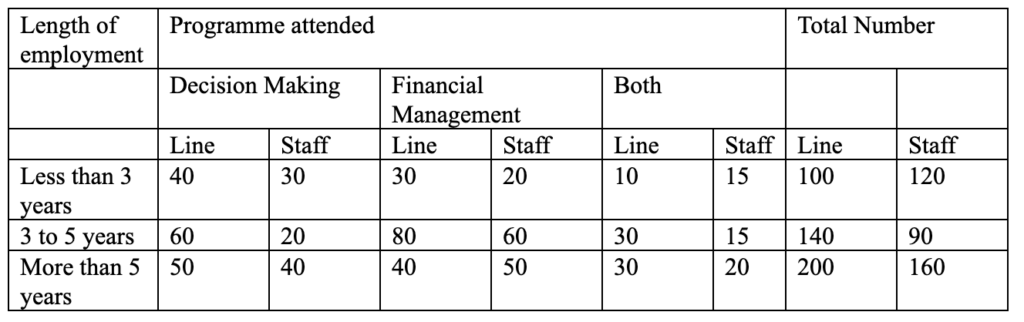Q1) What is the number of line employees with 3 to 5 years of employment who have not attended the program on Financial Management?

1. 190
2. 140
3. 60
4. 80

A1) Correct answer is C

Should be a straight fwd question for most of you.. Total no of line employees with 3-5 years of employment = 140 no. of line employee with 3-5 years of employment who attended financial management programme = 80 no of line employee with 3-5 years of employment who have not attended financial management programme = 140-80 = 60

Q2) What percent of the employees with more than 5 years of employment did not attend either workshop? (Select the value nearest to the correct value)

1. 64%
2. 86%
3. 50%
4. 14%

A2) Correct answer is (a)

Total no of employee with more than 5 years of employment = 360, no of employee with more than 5  years of employment who attended decision making programme = 90 no of employee with more than 5 years of employment who attended financial management programme = 90 no of employee who attended both the programme = 50 therefore no of employee who does not attended either programme = 360-(90 + 90 – 50 ) = 230. 230 / 360 =64%

Q3) How many of the employees who attended at least one program have more than 5 years of an employment?

1. 180
2. 130
3. 230
4. 36

A3) Correct answer is (b)

Total no of employee with more than 5 years of employment = 360. no of employee with more than 5 years of employment who attended decision making programme = 90 no of employee with more than 5 years of employment who attended financial management  programme = 90 no of employee who attended both the programme = 50 no of employee who attended at least one programme = ( 90 + 90 – 50 ) = 130

For questions 6 – 10, refer to the information below and then answer the questions

NUTRITIONAL VALUE OF SOME DAIRY PRODUCTS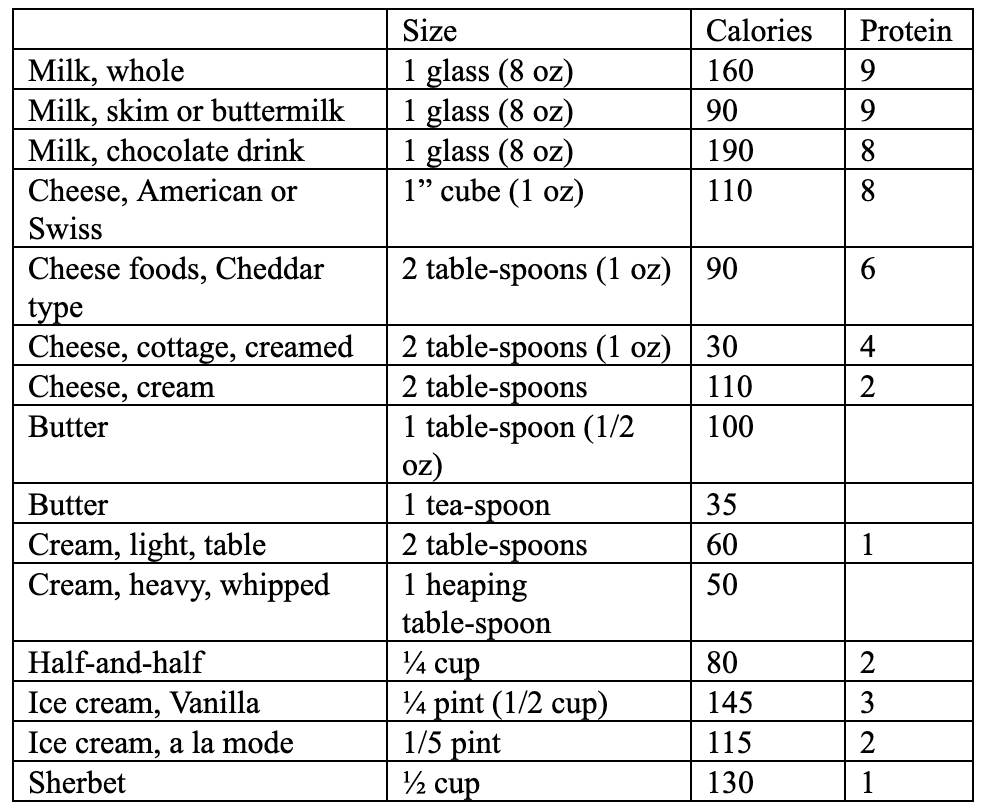Q4) Which of the following has the smallest number of calories per ounce ?

1. Cottage Cheese
2. Swiss Cheese
3. Butter
4. Whole Milk

A4) Correct answer is D

A) cottage cheese = 30 calories;

(B) cheddar – type cheese = 90 calories.

(C) Swiss cheese = 110 calories;

(D) butter = 200 calories;

(E) whole milk =20 calories

Q5) Which of the following furnishes the greatest number of grams of protein per unit weight ?

1. Whole Milk
2. Cottage Cheese
3. Butter
4. American Cheese

A5) Correct answer is C

In 8 ounces, the number of grams of protein is: whole milk, 9 grams; cottage cheese, 32 grams; Butter, none; chocolate milk, 8 grams; American cheese, 64 grams.

Q6) In which of these scenarios does a person consume maximum number of calories ?

1. 1 glass of chocolate milk and 2 cups of vanilla ice cream
2. 1 glass of chocolate milk,3 cubes of swiss cheese and 1 cup sherbet
3. 1 glass of skim milk and 2 tsp of light cream
4. 2 glasses of whole milk and 1.5 cup of vanilla ice cream

A6) Correct answer is (a)

a) 1 glass of chocolate milk and 2 cups of vanilla ice cream (190 + 580 = 770)

b) 1 glass of chocolate milk,3 cubes of swiss cheese and 1 cup sherbet (190 + 220 + 260 = 670)

c) 1 glass of skim milk and 2 tsp of light cream (90 + 60 = 150)

d) 2 glasses of whole milk and 1.5 cup of vanilla ice cream (320 + 435 = 755)

For questions 7- 10, refer to the information below and then answer the questions

Truck freight rates (in rupees) for various commodities from Delhi to various states (per 9 tonnes) are as follows:

 Andhra Pradesh Vishakhpatnam 19000 Assam Guwahati 26000 Tinsukhia 30000 Bihar Patna 16000 Ranchi 15000 Jharia 16500 Tata 16000 Gujarat Ahmedabad 6800 Surat 8000 Vadodara 7100 Kandla 8000 Haryana Panipat 2000 Karnal 2300 Yamunanagar 2350 Kaithal 2400 Ambala 2700 Sirsa 2800 Madhya Pradesh Bhopal 8000 Jabalpur 11000 Bilaspur 17000 Raipur 16000 Maharashtra Mumbai 11500 Pune 14000 Nagpur 10000 Kolhapur 14800 Karnataka Bangalore 20000 Mysore 20800 Punjab Amritsar 4200 Rajpura 3300 Chandigarh 3100 Jalandhar 4600 Bhatinda 4300 Khanna 3900 Rajasthan Jaipur 2700 Ajmer 3000 Alwar 2500 Udaipur 3600

Q7) Mr. Sharma is a renowned businessman of Delhi. He sent some trucks to Ajmer and some to Alwar. For this he paid Rs x. His average payment per trip was Rs. 2650. Had he sent one truck to Jharia and one to Nagpur he would have paid Rs. x then also. How many rupees he will pay as freight for the trips to Bhatinda? It is given that trips to Bhatinda was equal in number to the trips to Ajmer.

1. 30100
2. 17200
3. 12900
4. None of these

A7) Correct answer is (c)

Freight for one truck to Jharia = Rs. 16500 Freight for one truck to Nagpur = Rs. 10000

Total = Rs. 26500

Number of trucks sent to Ajmer and Alwar = 26500/2650 = 10

Ratio of trips to Ajmer and Alwar can be found out by allegation method as follows: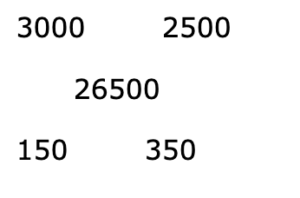= 150 : 350  = 3 : 7 Since total number of trips to Ajmer and Alwar is 10.

Therefore no. of trips of Ajmer = 3 = trips to Bathinda

Freight for three trips to Bhatinda = 3 × 4300 = Rs. 12900.

Q8) What is the difference between the average freight rate for all the given locations of Maharashtra and that of Haryana?

1. Rs. 10050
2. Rs. 11050
3. Rs. 10150
4. Rs. 12005

A8) Correct answer is (c)

Average Maharashtra = 50300/4 = 12575. Average Haryana = 14550/6 = 2425. Difference = 10,150

Q9) Mr. Sharma has a decision to make re selling 9,000 tonnes of commodities. He can either sell them in Maharashtra for Rs 2 crore or in Haryana, where they will be 10% cheaper. Considering other costs besides freight to be similar, which scenario allows him to make more profit ?

1. Selling in Maharashtra
2. Selling in Haryana
3. Same for both states

A9) Correct answer is (b)

Take only 2 known variables into account, Profit = Sales Prices – Freight and use the average freight information from previous question

Profit for Maharashtra = Rs 2 crore – Rs 1.26 cr = Rs 0.74 cr

Profit for Haryana = Rs 1.8 cr – Rs 1.02 cr = Rs 0.78 cr

Q10) If there is no selling price difference between Maharashtra and Haryana, then which scenario allows him to make more profit ?

1. Selling in Maharashtra
2. Selling in Haryana
3. Same for both states

A10) Correct answer is (b)

Profit for Maharashtra = Rs 2 crore – Rs 1.26 cr = Rs 0.74 cr

Profit for Haryana = Rs 2 cr – Rs 1.02 cr = Rs 0.98 cr

## DI – Practice Test 1

(questions relate to teaching material from DI 1)

For questions 1-5, refer to the information below and then answer the questions

For a particular company, the table below shows the monthly Income of employees from different sources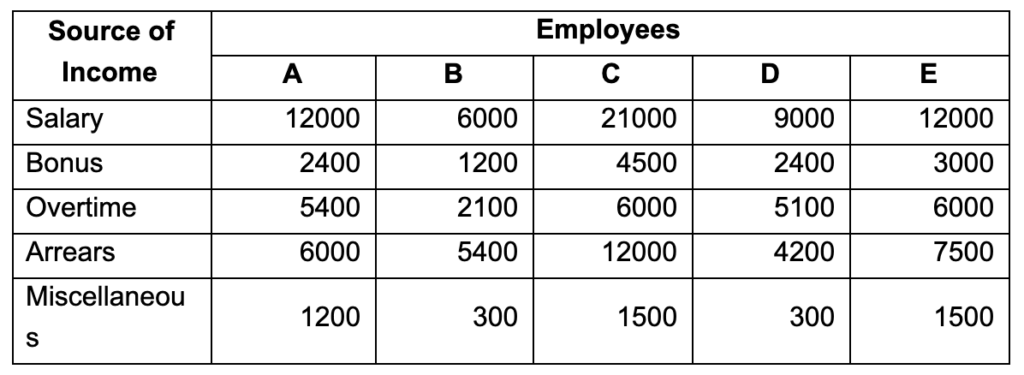1) Which of the following categories of employees has minimum ratio of arrears to income from salary?

1. A
2. B
3. C
4. D

A1) Correct answer is (d)

Except D, for all other employees, Arrears are equal to or more than 50% of salary. In case of D, Arrears are 4200/9000 = 46% of salary.

2) Which of the following categories of employees earn maximum bonus in comparison to their total income?

1. A
2. B
3. C
4. D

A2) Correct answer is (d)

In such cases, it is advisable to calculate total income upfront. The respective total incomes are A: 27,000; B: 15,000; C: 45,000; D: 21,000; E: 30,000. Now using 10% as benchmark, we find that only in case of D, bonus exceeds 10% of total income.

3) Which of the following categories of employees have the maximum % of their salary out of the total income?

1. A
2. C
3. D
4. E

A3) Correct answer is (b)

A: (12000/27000) = 44.44%. C: (21000/45000) 46.67%. D: (9000/21000) = 42.85%. E : (12000/30000 = 40%).

Hence, salary to total income ratio is maximum in case of C

4) How many categories of employees have their salary less than four times the income from bonus?

1. 0
2. 1
3. 2
4. 3

A4) Correct answer is (b)

Only D category employees have salary less than 4 times the income from bonus.

5) The income from overtime is what % of the income from arrears in case of category E?

1. 8
2. 25
3. 20
4. None of these

A5) Correct answer is (d)

Required percentage = 6000/7500 = 80%

For questions 6- 10, refer to the information below and then answer the questions

The following table shows the marks obtained by some students in an examination. The number within brackets shows the maximum marks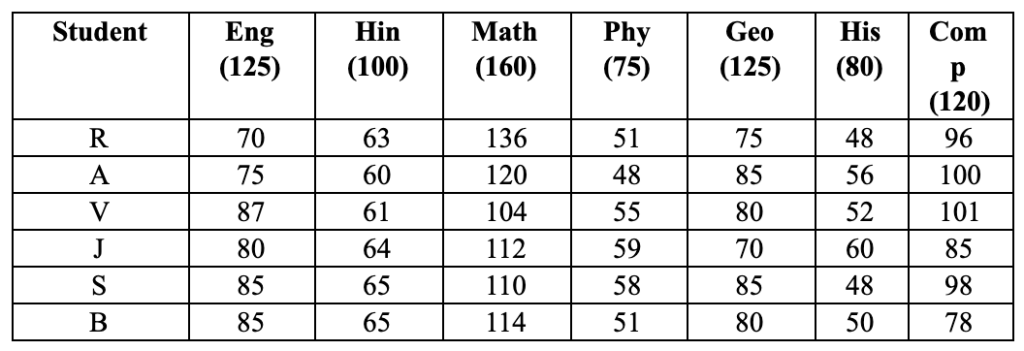6) Percentage of marks obtained by V in Maths and History together is equal to the percentage of marks obtained by B in which of the following subjects together?

1. English, Geography
2. Physics, History
3. Hindi, Computer
4. Math, Geography

A6)

For V, Maths & History % = (104+52)/(160+80) = 156 / 240 = 65%

For B,optio

n C leads to 65%  (65 + 78) / (100+120) = 143 / 220

7) How many students have secured 60% or more marks in each subject?

1. Two
2. One
3. Three
4. Four

A7) Correct answer is (d)

Except for R (Emglish) and J (Geography), the other four students have scored

greater than 60% in each subject

8) Find the difference in the percentage marks obtained by R in Geography to that

by J in Maths.

1. 37%
2. 70%
3. 15%
4. 10%

A8) Correct answer is (d)

R (Geography) = 75 / 125 = 60%

J (Maths) = 112 / 160 = 70%

9) For the two language subjects, English and Hindi, the ranking of students in decreasing order of their percentage scores is

1. RAVJSB
2. BSJVAR
3. JVASBR
4. JARBVS

A9) Correct answer is (b).

No need to calculate % here since the denominator is same for all. Just add their English and Hindi scores

R – 133    A – 135    V – 148    J – 144        S – 150    B – 150

10) The person with strongest aptitude for engineering (as shown by their scores for Maths, Physics and Computers is)

1. R
2. A
3. V
4. B

A10) Correct answer is (a) -> R

Just add the Maths, Physics and Computers score for all

R – 283    A – 268    V –  285    J – 256        S – 266    B – 243

DI – 2

## Graphs & Charts

Graphs and Charts are drawings that show the relationship between changing things.

They are a diagram displaying the relationship between numbers or amounts. Common graphs use bars, lines, or parts of a circle to display data (pie chart)

### DI 2.1 Line Graphs

Line graphs compare two variables. Each variable is plotted along a vertical axis and a horizontal axis. So, for example, if you wanted to see the stock price variation of BSE Sensex (Bombay Stock Exchange) you can put time along the horizontal, or x-axis and stock price along the vertical, or y-axis and get the line graph as shown below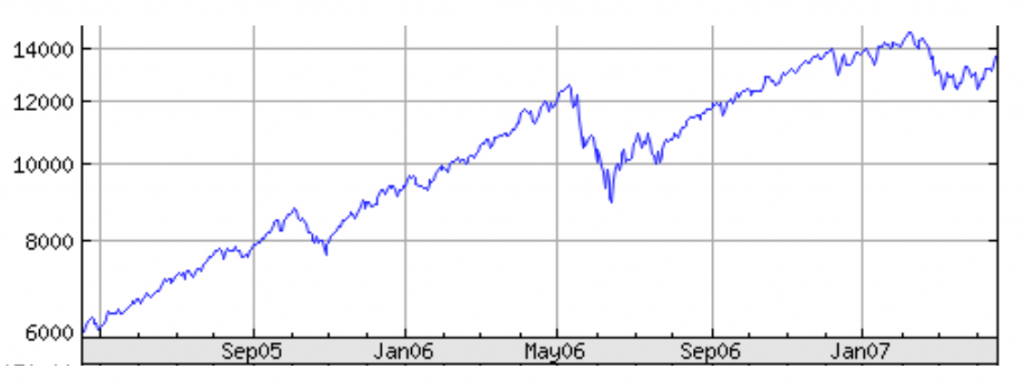Some of the strengths of line graphs are that:

• They are good at showing specific values of data, meaning that given one variable the other can easily be determined.
• They show trends in data clearly, meaning that they visibly show how one variable is affected by the other as it increases or decreases.
• They enable the viewer to make predictions about the results of data not yet recorded.

At the same time, line Graphs have a strong negative factor. It is possible to alter the way a line graph appears to make data look a certain way. This is done by either not using consistent scale on the axes (meaning that the value in between each point along the axis may not be the same) or when comparing two graphs using different scales for each. It is important to be aware of this as you visually try to make inferences from graphs.

For questions 1 – 5, refer to the information below and then answer the questions

For a particular retail store X, the graph below shows the weekly variation of “Average Daily Amount of Sales” and “Number of Sales” (all numbers are in thousands)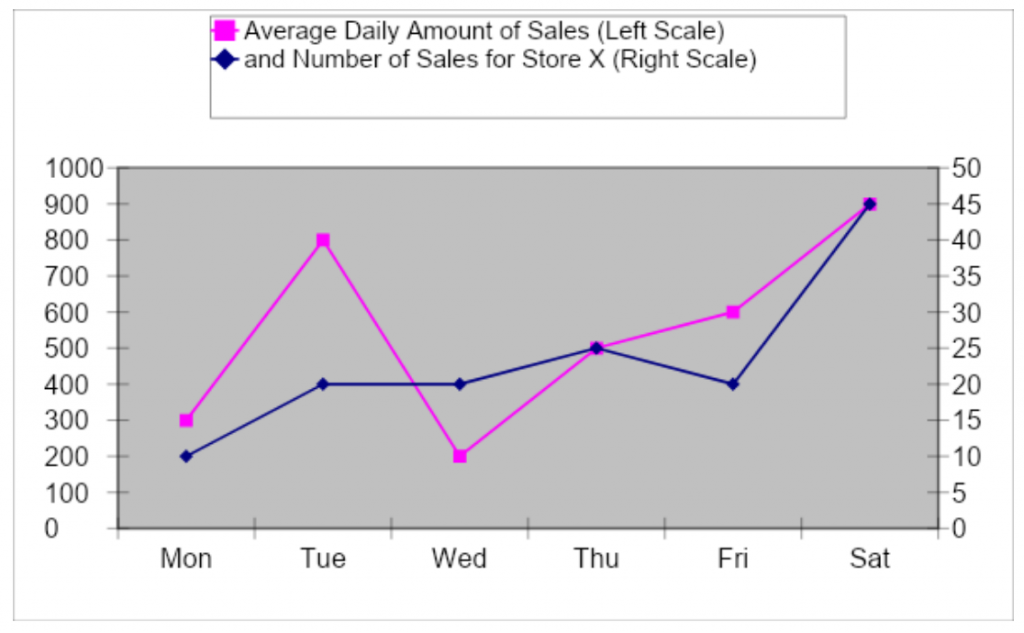Q1)  Total amount of sales averaged for the given number of days is:

1. Rs 600
2. Rs 650
3. Rs 700
4. Rs 550

A1) Correct answer is (d)

Required average = (300 + 800 + 200 + 500 + 600 + 900)/6 = 550

Q2) Number of days on which the total sales are less than the average sales for the given period is:

1. 3
2. 2
3. 4
4. 6

A2) Correct answer is (c)

As you can see from Q1, there are 3 days in which average sales are less than 550

Q3) The maximum value of the average daily amount of sales per sale is:

1. Rs 20
2. Rs 30
3. Rs 25
4. Rs 40

A3) Correct answer is (d)

The average daily amount of sales per sale is calculated for various days as Mon: 300/10; Tue: 800/20; Wed: 200/20; Thu: 500/25; Fri: 600/20; Sat: 900/45. You can see, it is maximum at Rs 40 on Tuesday

Q4) Opening balance of cash at the sales counter on Monday was Rs 1,000. During the week, expenses of Rs 800 were paid through the counter. The closing balance of cash on Saturday was:

1. 2500
2. 3000
3. 3500
4. 2000

A4) Correct answer is (c)

1,000 + (550×6) – 800 = 3,500

Q5) If Median is defined as the average of two mid values when arranged in ascending order, the median amount of sales per median number of sales for the given period is:

1. 25
2. 27.5
3. 30
4. 32

A5) Correct answer is (b)

Amount of sales in ascending order: 200, 300, 500, 600, 800, 900.Median amount of sales: (500 + 600)/2 = 550

No. of sales in ascending order: 10, 20, 20, 20, 25, 45. Median no. of sales: (20 + 20)/2 = 20

Median amount of sales per median no. of sales = 550/20 = 27.50

For questions 6 – 8, refer to the information below and then answer the questions

Given are the price movements of four commodities cardamom, pepper, sugar, and gold over the weeks from January to April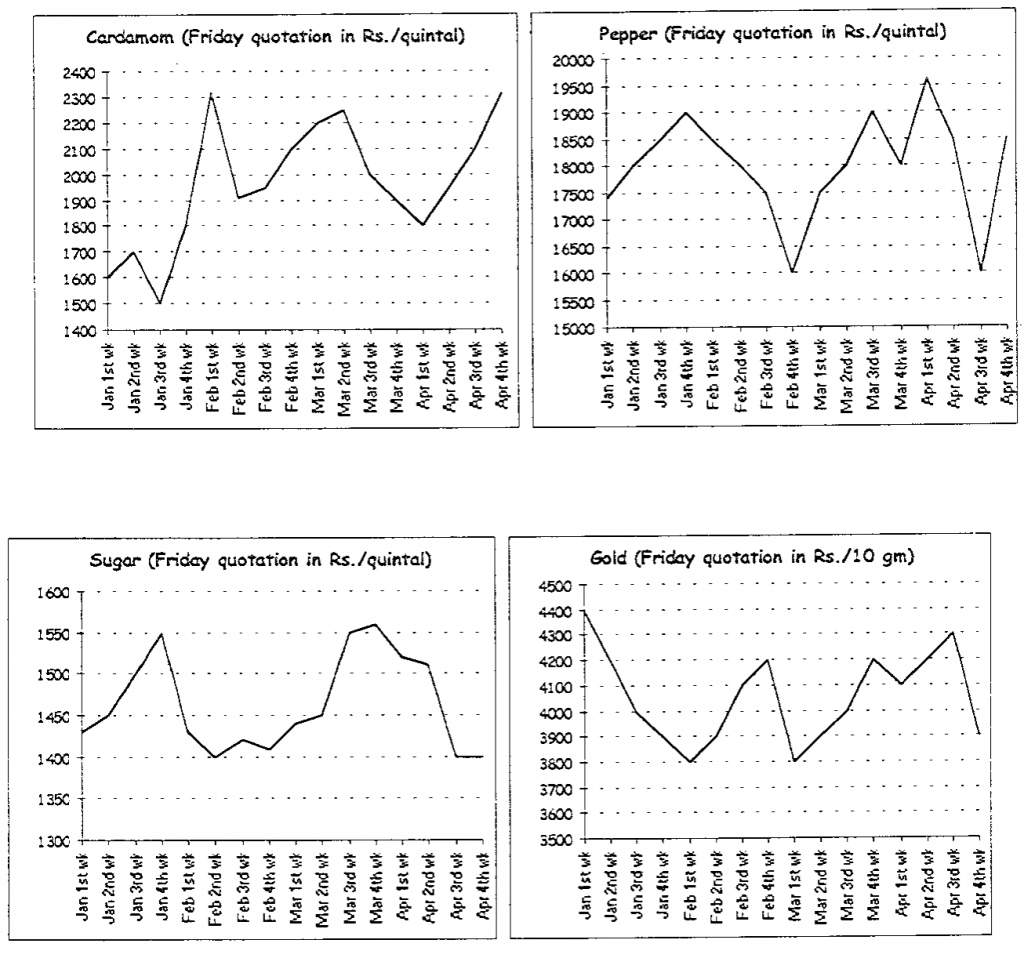Q6) The price change of the commodity is the absolute difference in the ending and beginning prices expressed as a percentage of the beginning price. Which commodity has the highest price change?

1. Cardamom
2. Pepper
3. Sugar
4. Gold

A6) Correct answer is (a)

Price Change for Cardamon = (2300 – 1600) / 2300 = 44%. Others are lower than this

Q7) Price volatility is equal to highest price minus lowest price divided by average price during the period. Which commodity has the lowest price volatility?

1. Cardamom
2. Pepper
3. Sugar
4. Gold

A7) Correct answer is C

You can do calculations for each commodity or visually see that the variation between highest and lowest price is minimum for sugar

Q8) X invested 25% in each of the four commodities in the beginning of the period and sole in the end. His investment resulted in

1. 10% profit
2. 5.5% loss
3. No Profit No Loss
4. 4.3% profit

A8) Correct answer is (a)

 Beginning End Increase Cardamon 1600 2300 44% Pepper 17500 18500 6% Sugar 1400 1440 3% Gold 4400 3900 -11% Average 10%

For questions 9 – 12, refer to the information below and then answer the questions

Mr. Malik went to US on a business trip and rented a car to drive from his hotel to the plant that he was visiting. Being new to driving in US, he was very cautious and wanted to ensure he drove within the speed limits. The graph below shows the speed he was driving at different times of the day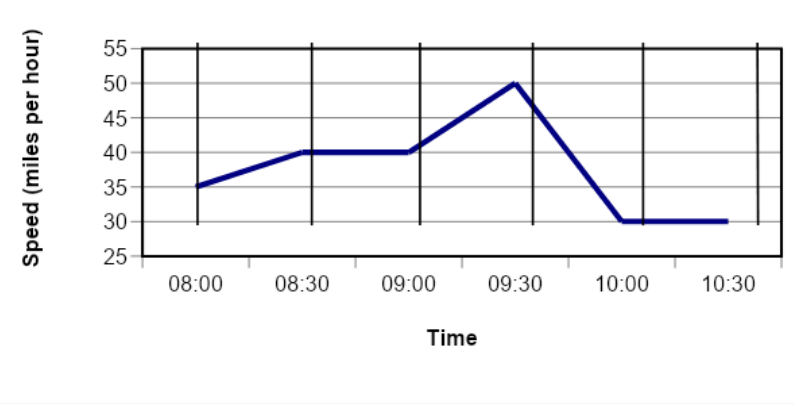Q9) For what percent of the time was he driving at 40 miles per hour or faster?

1. 20
2. 45
3. 40
4. 50

A9) Correct answer is (d)

A quick glance at the graph will show you that x axis has equally spaced intervals. Out of the 5 intervals that he drove, for 2.5 of those the graph on y axis is above 40, so 50%

Q10)    How far, in miles, did Mr. Malik drive between 8:30 and 9:00?

1. 0
2. 20
3. 30
4. 40

A10) Correct answer is (d)

Since his speed was 40 miles per hr, he would have driven 40 miles

Q11)    The total distance traveled by Mr. Malik is

1. less than 50 miles
2. between 50 and 100 miles
3. greater than 100 miles
4. Can’t be calculated

A11) Correct answer is (b)

Take the average speed for 30min. intervals and divided by 2 (since each interval is ½ hr)

( 37.5 + 40 + 45 + 40 + 30 )/2 = 192.5 / 2 = 96.125

Q12) How much time Mr. Malik would have taken if he had traveled the whole distance at the average speed of 40 miles per hr

1. 2 hrs
2. 2.8 hrs
3. 2.4 hrs
4. 3 hrs

Use data from previous question re distance traveled 96.125 / 40 = 2.4 hrs approx.

For questions 13 – 15, refer to the information below and then answer the questions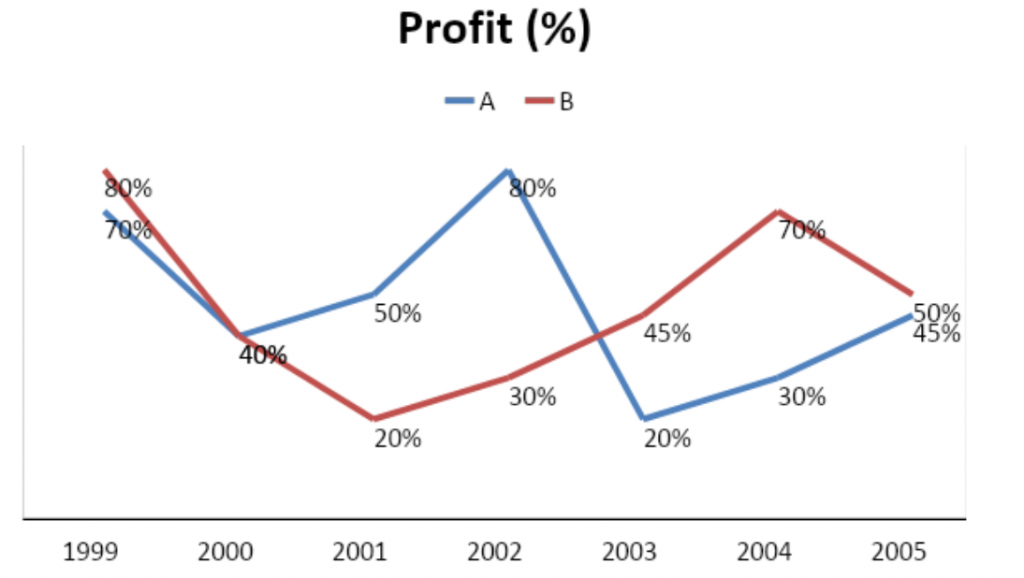Q13) If the total expenditure of company A and company B was Rs. 8 crores in the year 2000, then what was the total profit gained by both the companies together?

1. Rs. 6.4 cr
2. Rs. 1.6 cr
3. Rs 5.3 cr
4. None of these

Expense = Revenue *(1-Profit%) or 8 = R(1-40%) or R = 8/0.6 or P = 8*0.4/0.6 = 16/3

A14)  In which of the following years total profits of both the companies were equal?

1. 2000
2. 2005
3. Can’t say
4. None of these

We cannot say anything about the quantum of profits without knowing the revenue.

Q15  In the year 2002 it was observed that the ratio of the expenditure of the companies A and B was 3 : 8. Then the percentage profit earned by company A was what percentage of the profit earned by company B?

1. 100%
2. 266.66%
3. 166.66%
4. None of these

A15) Correct answer is (b)
Expenditure statement is more of a distraction than anything. Answer is 80%/30% =
266.66%

### DI 2.2 Bar Graphs

Bar graphs are used to display data in a similar way to line graphs. However, rather than using a point on a plane to define a value, a bar graph uses a horizontal or vertical rectangular bar that levels off at the appropriate level.

There are many characteristics of bar graphs that make them useful. Some of these are that:

1. They make comparisons between different variables very easy to see.
2. They clearly show trends in data, meaning that they show how one variable is affected as the other rises or falls.
3. Given one variable, the value of the other can be easily determined.

Bar graphs are an excellent way to show results that are one time, that aren’t continuous – especially samplings such as surveys, inventories, etc. For example, the graph below shows the Exit Polls / Opinion Poll for 2004 reelection for Bihar Assembly, based on survey conducted by different news groups. As you can see, its much better to see this information in a bar chart rather than a table or a line graph.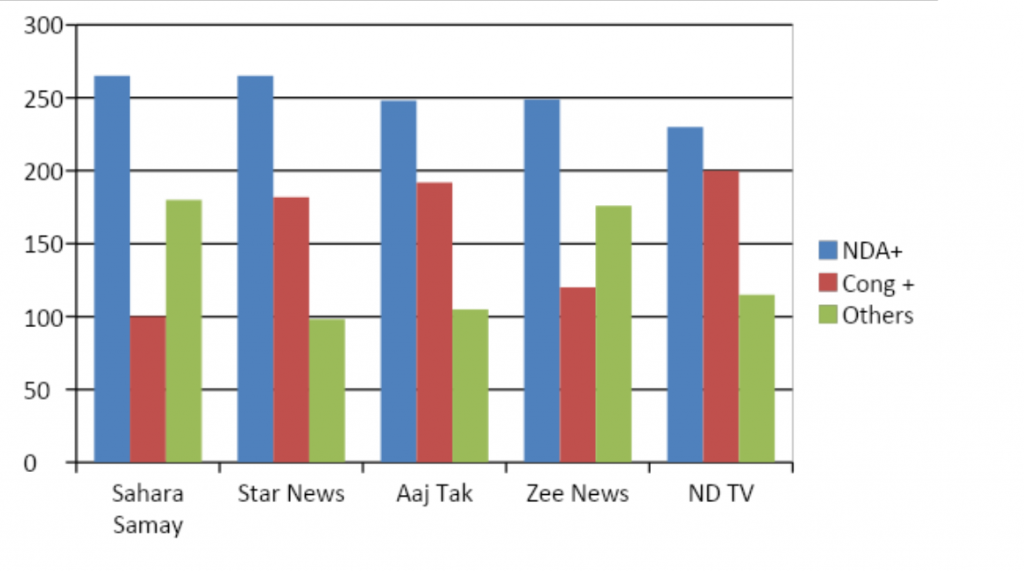Now lot of questions can be answered based on the graph

• Which political party is expected to win majority of the seats ?
• What is the expected margin between the 1st and 2nd place ?
• Would “Others” matter ?
• If all “Others” vote with Cong, would they have higher seats than NDA ?

Lets try this logic in some of the questions

For questions 1 – 3, refer to the information below and then answer the questions

The graph below shows how Revenue has changed from 2001 – 04 for 3 competitors in the same industry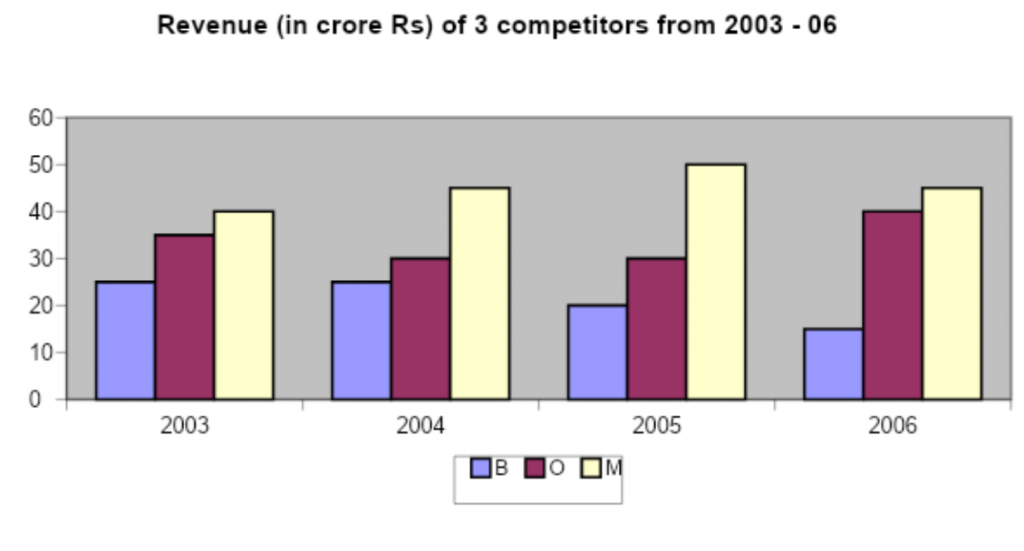Q1) If the three competitors together make up 100% of the industry, the year that saw the maximum percentage growth in the industry (as measured by turnover) was

1. 2003
2. 2004
3. 2005
4. 2006

A1) Correct answer is (b)

Revenue for each year is as shown below. Max. % growth from 2001 to 2002

2003 = 25 + 35 + 40 = 80

2004 = 25 + 30 + 45 = 100

2005 = 20 + 30 + 50 = 100

2006 = 10 + 40 + 45 = 95

Q2) For how many years did company M had majority share (50% or more) in the market

1. 0
2. 1
3. 2
4. 3

For years 2004 and 2005, M had 50% market share

Q3) The percentage increase in Revenue for Company ‘O’ from 2003 to 2006 is

1. < 10%
2. 10% – 20%
3. (10%) – (15%)
4. 20% – 50%

A3) Correct answer is (b)

(40-35) / 25 = 1/7 = 14.3%

For questions 4 -8, refer to the information below and then answer the questions

The graphs below show the year wise Exports, Imports, and Loans for a given country in Africa.  X, Y, Z and a, b, c are % components for exports and imports respectively

Composite Index = [% share/Loans taken for the specific years] x 100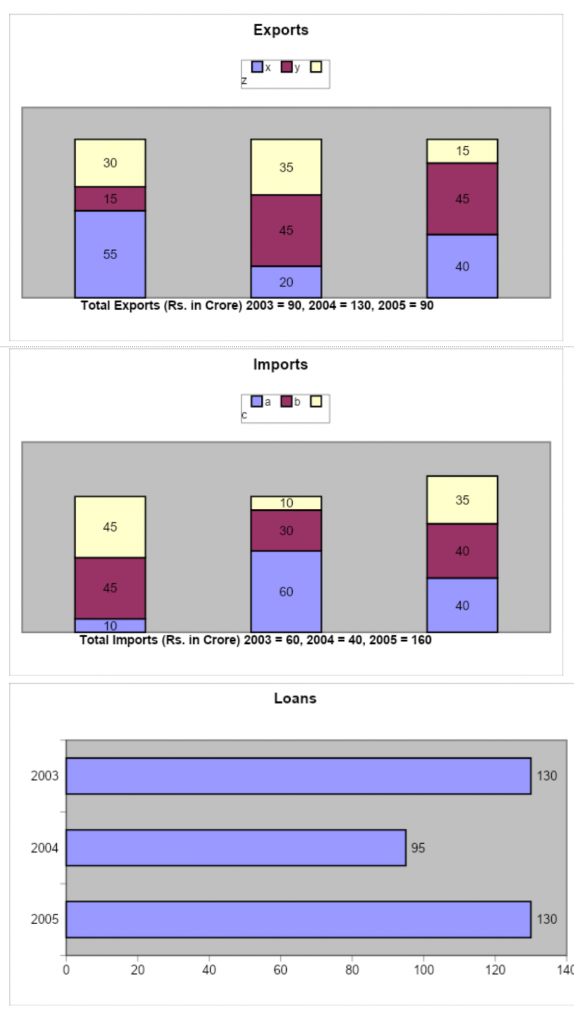Q4)  The average of value of X forms what % of the average value of Y over the 3 years’ period?

1. 76%
2. 99%
3. 110%
4. 82%

A4) Correct answer is (b)

The values of X over the three years are: 55% of 90, 20% of 130, 40% of 90.

The average value = {49.5 + 26 + 36}/3 = 37.16.

Similarly the average value of y = {58.5 + 13.5 + 40.5}/3 = 37.50

37.16/37.59  = 99.090%

Q5) The composite index for b in 2004 is what % of the same for X in 2005?

1. 36%
2. 79.42
3. 91%
4. 102.6%

A5) Correct answer is (d)

Composite Index for b in 2004= 30/95 = 31.57%

C.I. for X in 2005 = 40/130 = 30.76%

31.57/30.76 x 100 = 102.63%.

Q6) The average composite index for C, for the 3 years shown, is:

1. 24.01
2. 38.7
3. 13.6
4. 17.24

A6) Correct answer is (a)

Composite Index of c for 2003 = 45/130 x 100 = 34.61. For 2004 = 10/95 x 100 =10.52. For 2005= 35/130 x 100 = 26.92

Average value = {34.61 + 10.52 + 26.92}/3 = 24.01. Ans. (1)

Q7) The average value of Z forms what % of the average value of C for the given 3 years?

1. 110%
2. 79%
3. 98.8%
4. 28.72%

Value of Z in 2003: 0.30 x 90 = 27; 2004: 0.35 x 130 = 45.5; 2005: 0.15 x 90 = 13.5

Average value = 28.66. Similarly the average value of c = [27 + 4 + 56]/3 = 29

% = 28.66/29 x 100 = 98.82%

Q8) The average composite index for X, Y, Z in 2003 is greater than that in 2005 by about:

1. 13.69
2. 0%
3. 3%
4. 42.44%

A8) Correct answer is (b)

Average composite index for X, Y, Z in 2003: 1/3(100/130 x 100)

Average composite index for X, Y, Z in 2005: 1/3(100/130 x 100).

Hence % difference = 0.

### DI 2.3 Pie Charts

Wikipedia defines pie chart as a circular chart divided into sectors, illustrating relative magnitudes or frequencies. In a pie chart, the arc length of each sector (and consequently its central angle and are), is proportional to the quantity it represents. Together, the sectors create a full disk. A chart with one or more sectors separated from the rest of the disk is called an exploded pie chart. It is named for its resemblance to a pie which has been sliced.

The portion of a slice = (degrees of angle made by the slice) / 360 degrees

For example, a pie chart can clearly show the percentage of people who prefer cheese pizza, paneer pizza, or chicken pizza.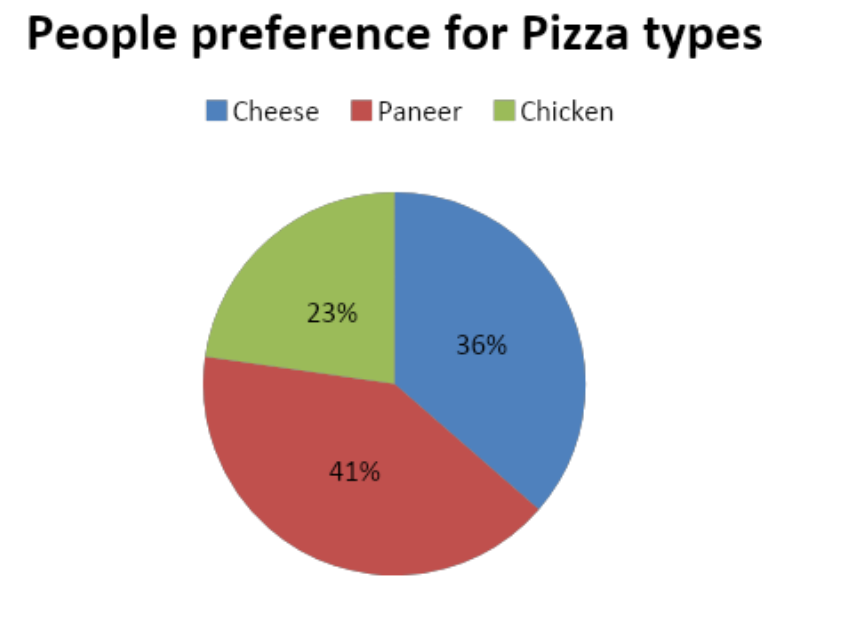Or we can take the same election data from the Bihar example shown earlier for Bar Charts. If the exit polls from Sahara Samay hold true, the assembly breakup will be as shown below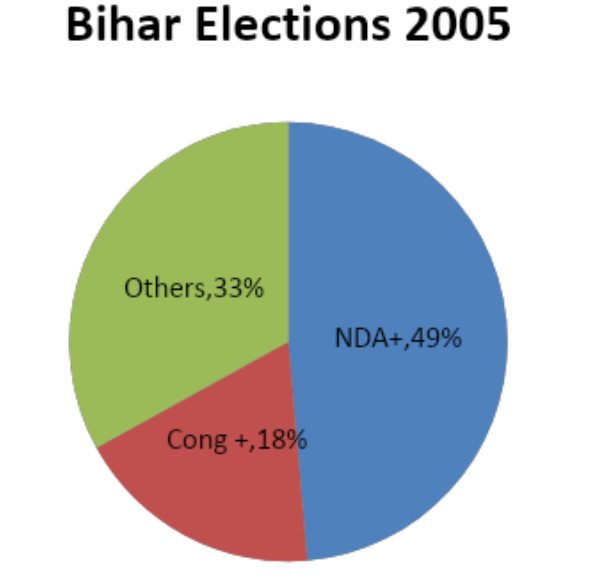For questions 1 – 5, refer to the information below and then answer the questions

The charts below show the breakup of debt position of India in years 1980 and 1985, when the total debt was Rs 2295 cr and Rs 4133 cr resp.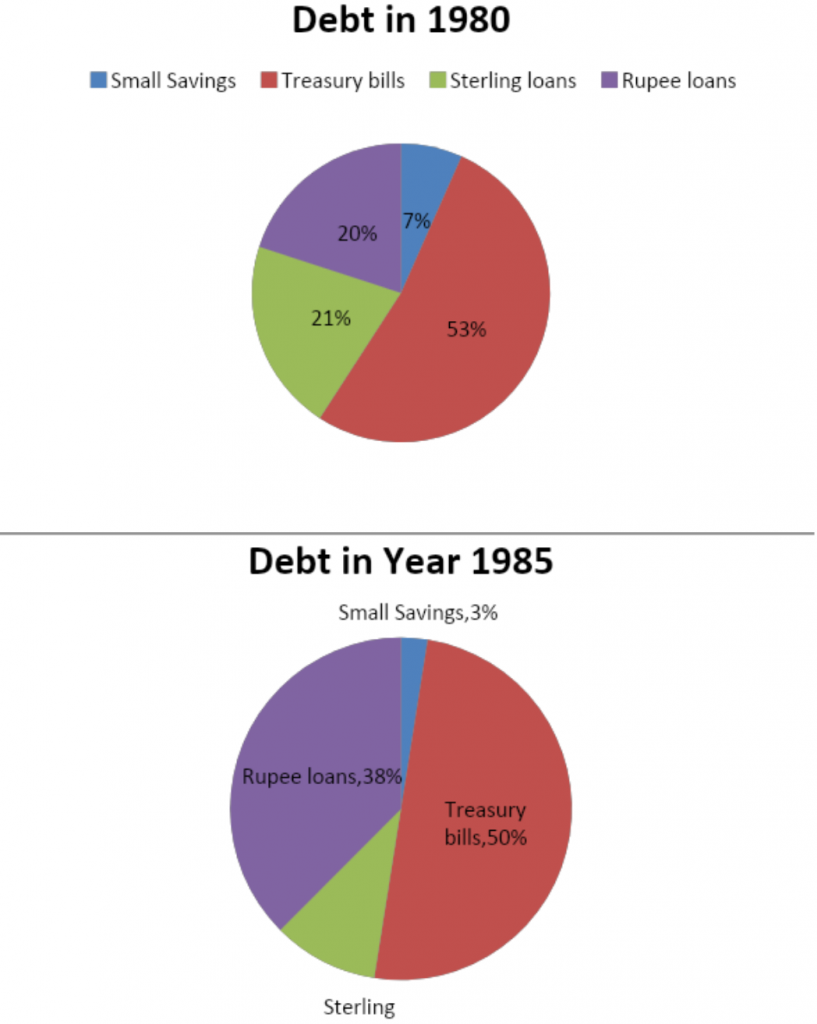Q1)  The item on which the debt is smaller during 1985 compared to 1980 is:

1. Treasury Bills
2. Small Savings
3. Sterling Loans
4. Small savings & Sterling Loans

A1) Correct answer is (d)

 1980 1985 Small Savings 153 103 Treasury bills 1,205 2,067 Sterling loans 478 413 Rupee loans 459 1,550

Q2)    The ratio of rupee loans of 1980 to that of 1985 is most nearly:

1. 3.3 : 1
2. 2.4 : 1
3. 1.2 : 3
4. 1 : 3

A2) Correct answer is (d)

(0.2 x 2295 / 0.38 x 4133)

Q3)    The item, whose proportionate debts form a ratio 3 : 2 between the two years is:

1. Rupee Loans
2. Small Savings
3. Sterling Loans
4. None of the above

A3) Correct answer is (b). See answer for Q1

Q4)    If the debt during 1980 increases three fold, the increase in debt on treasury bills from the present is most nearly:

1. 100%
2. 300%
3. 250%
4. 200%

A4) Correct answer is (d)

3 fold means 200% increase

Q5)    If the total debt for each of the two years is Rs. 1 lakh, the ratio of debt on sterling loans of 1980 to that of 1985 is most nearly:

1. 3 : 2
2. 1 : 2
3. 3 : 1
4. 2 : 1

A5) Correct answer is (d)

21/10  = 2 : 1 (approximately)

For questions 6 – 10, refer to the information below and then answer the questions

The charts below show profits and sales for a company over a period of 2001 – 2003. Use Expense = Sales – Profit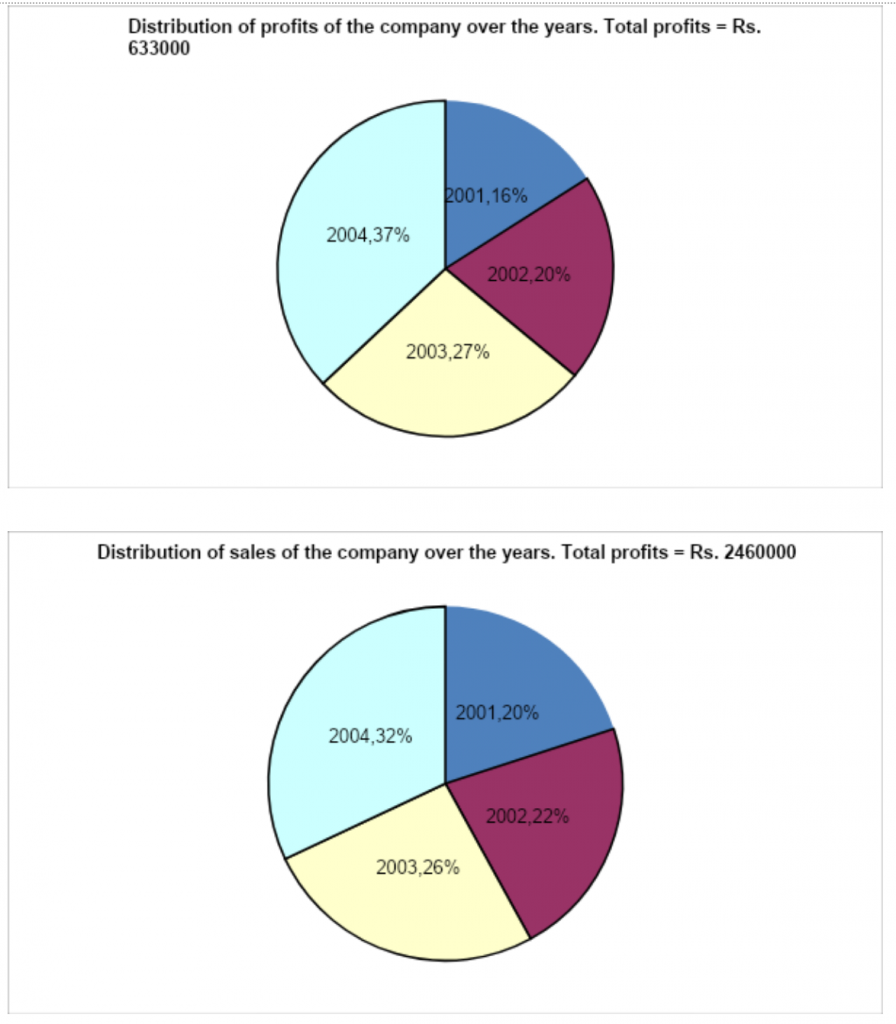Q6)  In which year are profits as a % of sales minimum?

1. 2001
2. 2002
3. 2003
4. 2004

A6) Correct answer is (a)

2001 = 100/500   2002 =  128/540

2003 =  170/630   2004 =  235/790

Q7)  In which year are profits as a % of sales maximum?

1. 2001
2. 2002
3. 2003
4. 2004

A7) Correct answer is (d)

Q8)    If % profit = Profit as a % of cost, % profit is maximum in:

1. 2001
2. 2002
3. 2003
4. 2004

A8) Correct answer is (d)

2001 = 100/400    2002 = 128/412

2003 =  170/460    2004 =  235/555

Q9)    % profit is minimum in (use earlier given definition of profit):

1. 2001
2. 2002
3. 2003
4. 2004

A9) Correct answer is (a)

Q10)    The average profit for the four years is what % of the average sales for the four years?

1. 126
2. 259
3. 196
4. None of the above

A10) Correct answer is (a)

Average profit = Total profit/Total Sales {63000/2460000} x 100 = 25.7%.

## DI – Practice Test 2

(questions relate to teaching material from DI 2)

For questions 1-5, refer to the information below and then answer the questions

For a particular company, the table below shows the performance for years 2000 – 2003 (all numbers in crores)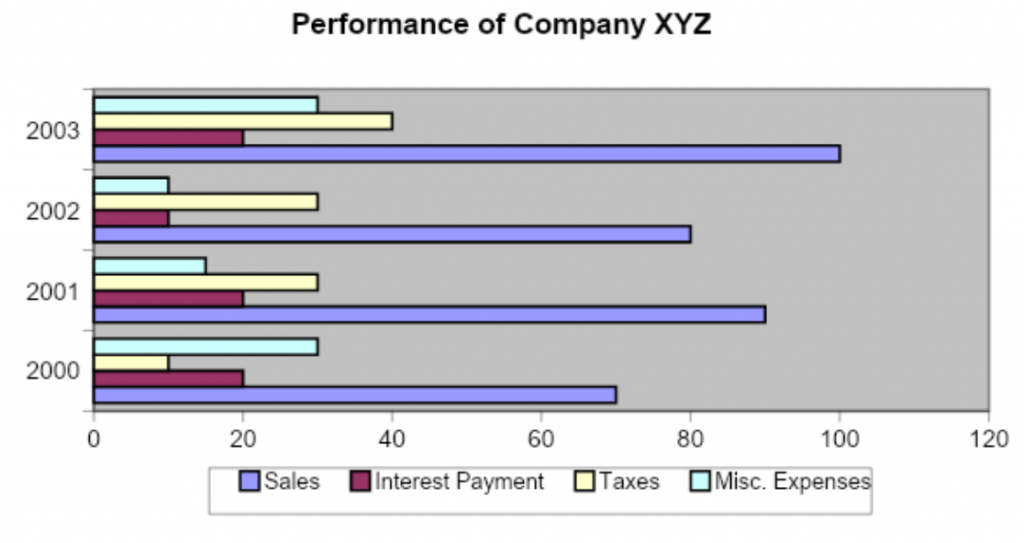1) The maximum value of profit is observed during the year:

1. 2000
2. 2001
3. 2002
4. 2003

A1) Correct answer is C

Profit = Sales – Interest Payments – Taxes – Misc. Expenses

2000: 70 – 20 – 10 – 30 = Rs. 10 crores

2001: 90 – 20 – 30 – 15 = Rs. 25 crores

2002: 80 – 10 – 30 – 10 = Rs. 30 crores

2003: 100 – 20 – 40 – 30 = Rs. 10 crores

2) The minimum value of Profit per rupee of Sales occurs during the year:

1. 2003
2. 2000
3. 2002
4. 2001

A2) Correct answer is (a)

2000 = 10/70, 2001 = 25/90, 2002 = 30/80, 2003 = 10/100

3) The total Interest Payment over the 4 years period shown is what percentage of total Sales during the same period?

1. 10%
2. 30%
3. 40%
4. None

A3) Correct answer is (d)

Total interest payments = 20 + 20 + 10 + 20 = Rs. 70 crores. Total sales = 70 + 80 + 90 + 100 = Rs. 340 crores. Required percentage = 70/340 = a value slightly greater than 20%. Ans. (4)

4) During which year is the sum of interest paid and taxes as a percentage of Profit the highest?

1. 2000
2. 2003
3. 2002
4. 2001

A4) Correct answer is (b)

2000 = (20+10)/10    2001 = (20+30)/25

2002 = (10+30)/30    2003 = (20+40)/10

5) During which year are the Miscellaneous Expenses as a percentage of Profit the lowest?

1. 2000
2. 2001
3. 2002
4. 2003

A5) Correct answer is C

The misc. expenses to profit ratio is greater than 1 in 2000 & 2003. It is 0.6 in 2001 and 0.33 in 2002. So the required ratio is lowest in 2002

For questions 6-10, refer to the information below and then answer the questions

India is undergoing a major boom in the retail industry. One mobile company had 3000 retail outlets in 2001, 4500 in 2002, 5500 in 2003 and 9000 in 2004. These outlets are divided into three categories – Small (S), Medium (M) and Large (L), depending on the size of the city they are in and the annual turnover that they have. The graph below shows the percentage breakup different types of retail outlets in those years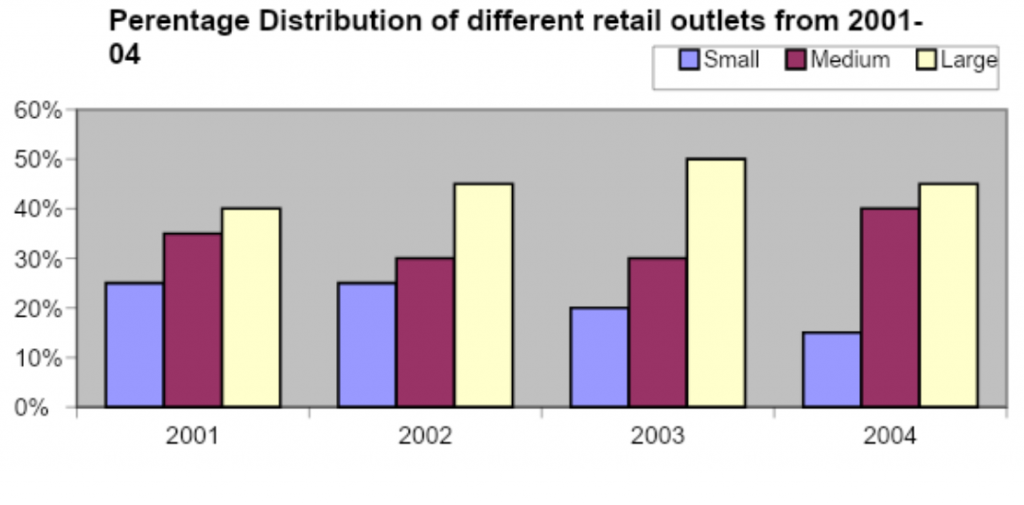6) The maximum percentage increase in the number of shops over the previous year is in:

1. 2001
2. 2002
3. 2003
4. 2004

A6) Correct answer is (d)

2002 = 15/30    2003 = 10/45    2004 = 35/55

7) The number of large size outlets in 2002 is more than the number of medium size

outlets in 2001 by:

1.  <1000
2. >1000
3. 1000
4. Can’t say

A7) Correct answer is (a)

Large size in 2002 = 4500 x 0.45 = 2025

Medium size in 2001 = 3000 x 0.35 = 1050

Difference is less than 1000

8) The number of which type of outlets (in %) is always highest, from 2001 – 2004) ?

1. Small
2. Medium
3. Large
4. Can’t say

A8) Correct answer is (c)

9) What is the percentage increase in the number of Medium size outlets from 2001 to 2004?

1. <200%
2. 300%
3. >200%
4. 100%

A9) Correct answer is (c)

Medium size in 2001 = 1050, in 2004 = 0.4 x 9000 = 3600. Growth > 200%

10) The number of small type shops in 2004 is what % of the number of large type in 2003

1. 50%
2. <50%
3. > 50%
4. None of the above

A10) Correct answer is (b)

Medium size in 2004= 0.15 x 9000 = 1350

Large size in 2003 = 5500 x 0.5 = 2750.

1350 / 2750 < 50%

For questions 11-20, refer to the information below and then answer the question

The graph below shows the sales (in million units) for 3 brands of a particular product of a FMCG company for years 2000 – 2005.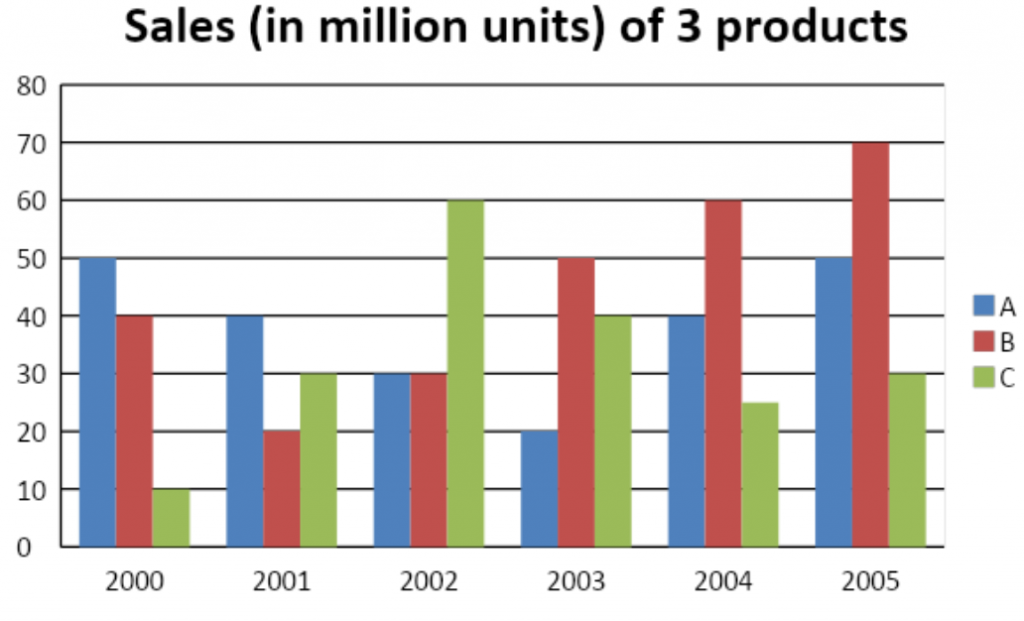Q11) In which of the following years was the sale of total of all the three brands taken together the lowest?

1. 2000
2. 2001
3. 2002
4. 203

A11)    Correct answer is (b)

The total sale of all 3 brands taken together, is given as:

For 2000, 10 + 40 + 50 = 100 units, For 2001, 20 + 30 + 40 = 90 units. For 2002, 30 + 30 + 60 = 120 units. For 2003, 20 + 40 + 50 = 110 units

Q12 ) In which of the following years was the sale of brand A & B exactly the same?

1. 2000
2. 2001
3. 2002
4. 2003

Rather simple question, can be easily answered by a visual of the graph

Q13) In which of the following years is the sale of brand C equals one-fourth of that of brand B?

1. 2000
2. 2001
3. 2002
4. 2003

A13)  Correct answer is (a)

In 2000, the sale of brand C equals one-fourth of that of brand B. (10 is one-fourth of 40)

Q14) The sale of brand A in 2002 was what percent of sale of brand B in 2004?

1. 25%
2. 100%
3. 75%
4. 50%

A14) Correct answer is (d)

30% for A in 2002 / 60% for B in 2004

Q15 ) The percent decrease in sale of brand C in 2004 as compared to 2003 is:

1. 15%
2. 25%
3. 40%
4. 37.5%

A15) Correct answer is (d)

Percent decrease = [(40 – 25) 40] x 100% = 37.5%.

Q16)  In case of how many years the sales of all three brands  has shown rise?

1. 1
2. 2
3. 3
4. none

A16) Correct answer is (a)

Happened only once, in 2005

Q17)  The ratio of sales of brand C to the combined figures of sales of brand A & B for 2002 is:

1. 1 : 2
2. 2 : 1
3. 1 : 3
4. 1 : 1

A17) Correct answer is (a)

60 : (30+30) = 1:1

Q18)  The ratio of the highest figure to the lowest figure, considering all three brands separately, is the highest for which of the following brands?

1. A only
2. B only
3. C only
4. Both B & C

The ratio of the highest figure to the lowest figure is given as: For brand A = 50 20 = 2.5, For brand B = 70 20 = 3.5, For brand C = 60 10 = 6.

Q19)  For the period shown, brand B registered an overall growth of:

1. 30%
2. 40%
3. 70%
4. 75%

A19) Correct answer is (d)

[(70 – 40) 40] x 100% = 75%.

Q20)  For the period shown, the overall percentage increase in sales for all 3 brands put together from 1986 to 1991 is:

1. 100%
2. 50%
3. 30%
4. 25%

A20) Correct answer is (b)

(150-100)/100 = 50%

## DI – Practice Test 3

(questions relate to teaching material from DI 2)

For questions 11-14, refer to the information below and then answer the questions

The graph below shows the percentage of students getting more than passing marks in a school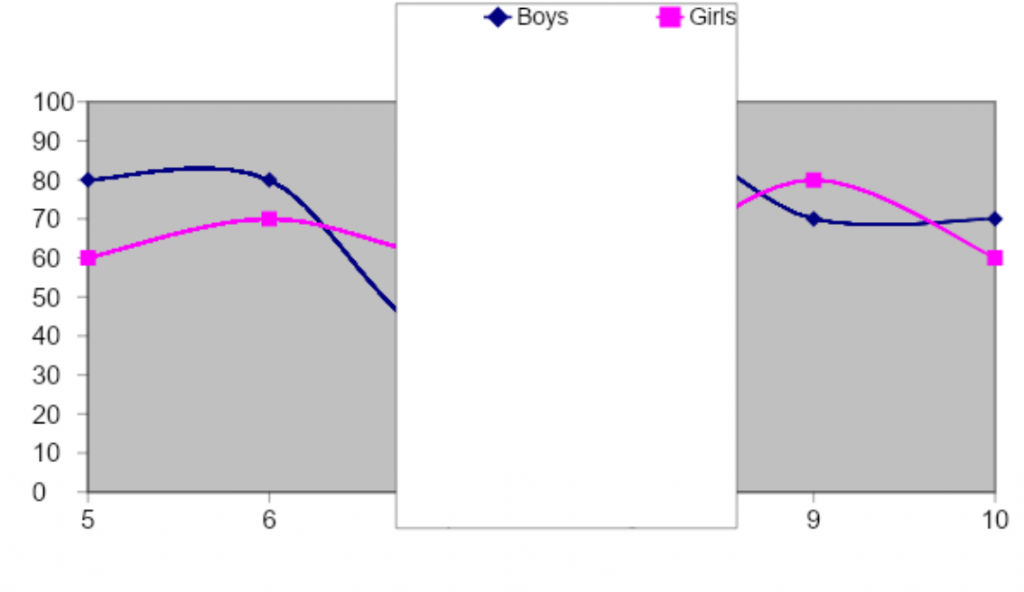1)    If the number of girls who passed in standard 5th is same as number of boys who passed in standard 5th, what is the ratio between the no. of boys  & the no. of girls in the standard 5th?

1. 4 : 3
2. 3 : 4
3. 3 : 8
4. 8 : 3

A1) Correct answer is (b)

Ratio is 60% to 80%

2)    If the overall percentage of passes in standard 7th is 46, and the total no. of boys is 200, what is total no. of girls?

1. 56
2. 66
3. 86
4. 106

A2) Correct answer is (c)

Let there be X girls in standard 7th. Then the overall pass percentage is given by (200 x 0.4 + X x 0.6)/(200 + X) = 0.46. Solving, we get X = 86 approximately.

3)    If the total no. of boys in each standard is 150 and number of girls 120, what is the overall % of passes in the school?

1. 69
2. 59
3. 49
4. 39

A3) Correct answer is (a)

There are 6 classes in all. Total no. of students = 6 x (150 + 120) = 1620.

Overall pass % = 150(0.8 + 0.8 + 0.4 + 0.9 + 0.7 + 0.7) + 120(0.6 + 0.7 + 0.6 + 0.6 + 0.8 + 0.6)/1620 = (150 x 4. 3 + 120 x 3.9)/1620 = 69% approximately.

4)    If the ratio between the no. of boys and the no. of girls in standard 9th is 4 : 1, what is the ratio between the number of boys passed & number of girls passed?

1. 5 : 11
2. 7 : 2
3. 11 : 5
4. 11 : 2

A4) Correct answer is (b)

Let there be 400 boys and 100 girls in Std. 9th. Then, no. of boys passing = 0.7 x 400 = 280.No. of girls passing = 0.8 x 100 = 80. Required ratio = 280 : 80 or 7 : 2.

For questions 5-14, refer to the information below and then answer the questions.

The graphs below show the monthly expenses for two families A and B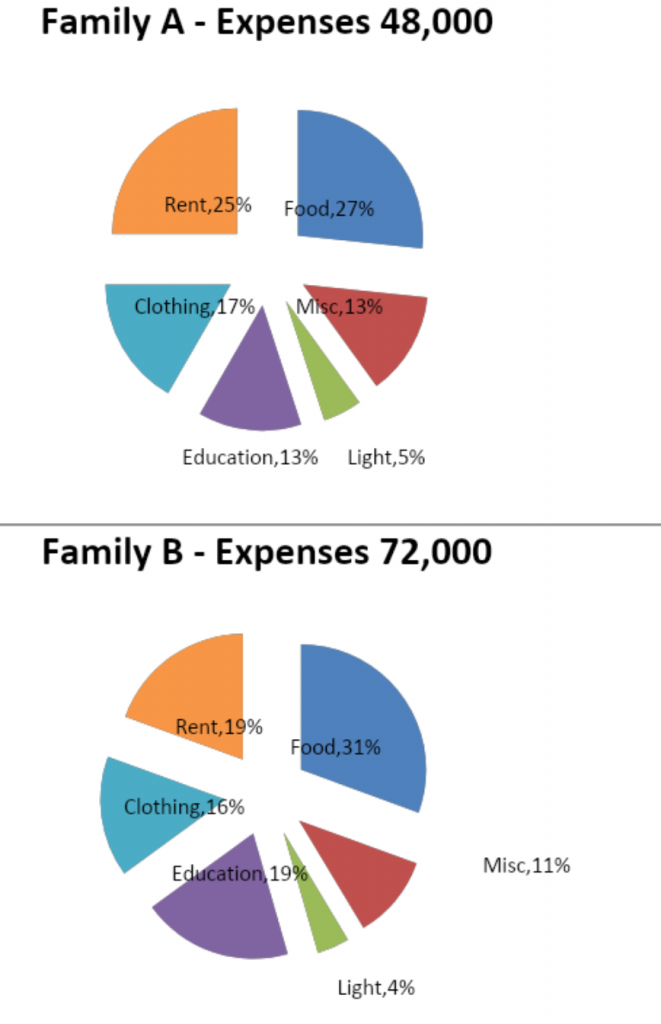Q5)    If the total expenditure of the two families is respectively doubled, then the ratio of expenses on “light” would be:

1. 1 : 1
2. 4 : 5
3. 2 : 3
4. 5 : 4

A5) Correct answer is (b)

Doubling would have no effect on the ratios. Family A spends 0.05x 4800 = 2.,400 on light, while Family B spends 0.15 x 72,000 = 3,000 on light. The ratio is 4:5

Q6 )Which of the following statements is true?

1. Total expenditure of A and B form a ratio 3 : 2
2. Family A’s expenditure on Rent is greater than that of B
3. Family A spends Rs. 6,400 on miscellaneous items
4. Family B spends less on light than family A

A6) Correct answer is (c)

Family A spends 0.13 x 48,000 = 6,400 on miscellaneous items

Q7)     If the expenditure of Family B increases threefold, the expenditure on education would be:

1. 20%
2. 28%
3. 2 fold nearly
4. can’t be determined

A7) Correct answer is (d)

You can’t assume proportionate increase in each of the individual expense items

Q8)    If the total expenditure of family A doubles and assuming proportionate increase for all categories, the difference between the expenditure of the families on Rent would be:

1. Rs. 1000
2. Rs. 10,000
3. Twice as it is now
4. None of the above

A8) Correct answer is (b)

Family A’s expense on rent will now be 24,000 while family B would be 14,000, a difference of 10,000

Q9)  If the radii of the circles are taken in the ratio of the square roots of 10 times the total expenditure of the families, then the radii of A and B form a ratio which is most nearly:

1. √2 : √3
2. 6 : 7
3. 7 : 9
4. √3 : 2

A9) Correct answer is (a)

√(480,000) : √(720,000) = √4 : √6 = √2 : √3

Q10)    The percentage of the expenditure on miscellaneous by family A over that of family B is:

1. less than 75%
2. Less than 80%
3. more than 80%
4. 80%

A10) Correct answer is (c)

6,400 by family A vs 7,800 by family B

Q11)   The item whose proportionate expenditure bears 1 : 1 ratio with some other item in family A and family B is:

1. Rent
2. Miscellaneous
3. Education
4. Light

A11) Correct answer is (c)

Family A, Education and Miscellaneous are same

Family B, Education and Rent are same

Q12) The item showing the least difference in expenditure between the two families is:

1. Food
2. Light
3. Clothing
4. Miscellaneous

A12) Correct answer is (b)

2,400 for family A vs 3,000 for Family B, a differnce of Rs 600. Other items have higher differences

Q13) In family A, the items which their expenditure showing 1 : 2 ratio are:

1. Education and Food
2. Food and Miscellaneous
3. Light and Rent
4. None of the above

A13) Correct answer is (a)

Education 13% vs Food 27%

Q14) The percentage expenditures of families A and B on food form a ratio:

1. 55 : 48
2. 4 : 7
3. 5 : 4
4. 45 : 52

A14) Correct answer is (d)

27 % : 31%. Mutiply both numerator and denominator by 2/3 and you get (d)

``` (adsbygoogle = window.adsbygoogle || []).push({}); ```

Jobs By Batch
Jobs By Location
Jobs By Degree
Jobs By Branch
IT Jobs
Internships
Govt. Jobs

``` (adsbygoogle = window.adsbygoogle || []).push({}); ```

• ##### Zoho off campus drive 2022/ 2023 | Multiple Hirings | Any Graduates/ Post Graduates, BE, B.Tech, MBA, MCA, ME, M.Tech | 2019, 2020, 2021, 2022, 2023 Batch | Multiple Location

``` (adsbygoogle = window.adsbygoogle || []).push({}); ```

• ##### Original Questions of IT Companies| All Topics

``` (adsbygoogle = window.adsbygoogle || []).push({}); ```